# Lateral Area Surface Area and Notes Lateral Area

• Slides: 10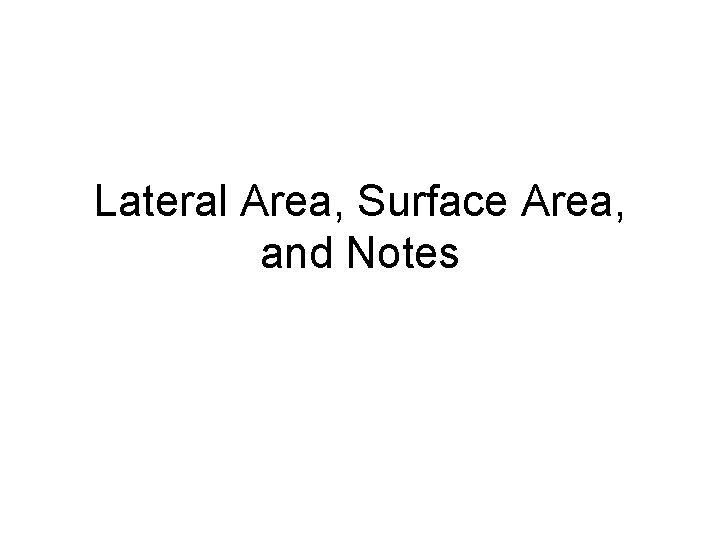Lateral Area, Surface Area, and Notes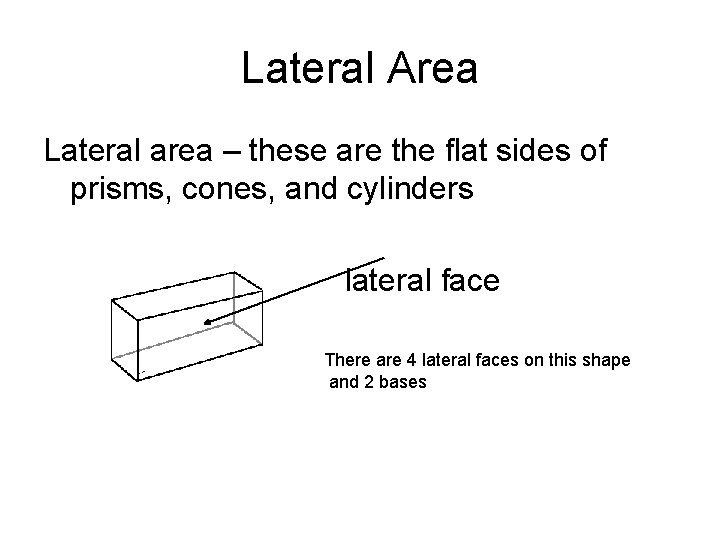Lateral Area Lateral area – these are the flat sides of prisms, cones, and cylinders lateral face There are 4 lateral faces on this shape and 2 basesDefinitions Lateral area – for prisms, cylinders and cones – the area of the figure not including the bases Surface Area – the sum of the area of all of the faces (sides and both bases) Volume – a measure of the amount of space contained in the interior of a solidPrisms To find Lateral Area: perimeter times height of prism LA = Ph 6 P is the perimeter of the base 4 h is the height of the prism 10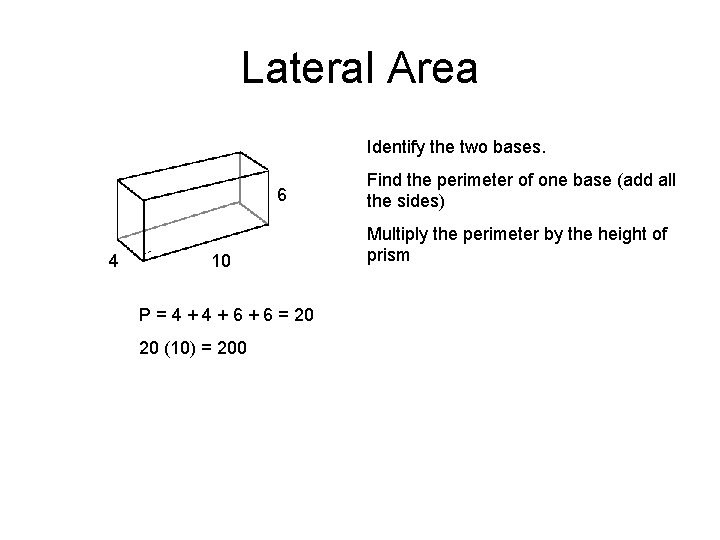Lateral Area Identify the two bases. 6 4 10 P = 4 + 6 + 6 = 20 20 (10) = 200 Find the perimeter of one base (add all the sides) Multiply the perimeter by the height of prism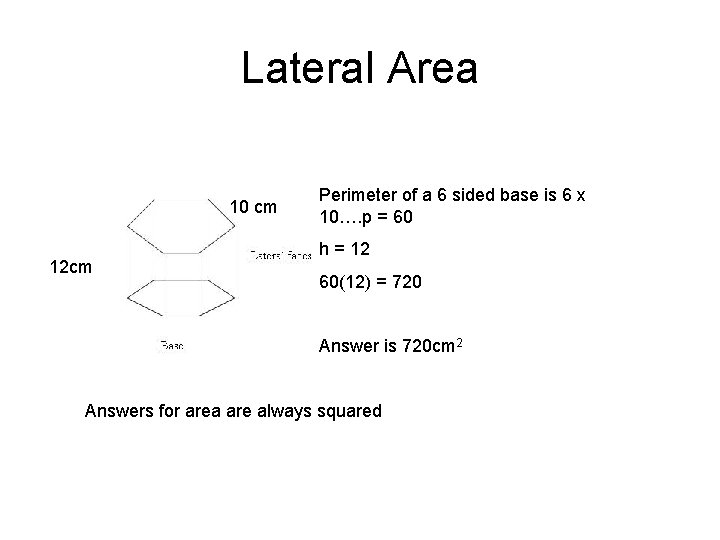Lateral Area 10 cm 12 cm Perimeter of a 6 sided base is 6 x 10…. p = 60 h = 12 60(12) = 720 Answer is 720 cm 2 Answers for area are always squaredSurface Area SA = LA + area of the 2 bases You need to know how to find perimeter of any polygon! (Just add all the sides) Steps: 1. Find P and h 2. Find LA 3. Find base area 4. Add 2 base areas and the LA to get SA 5. Final answer will be in units 2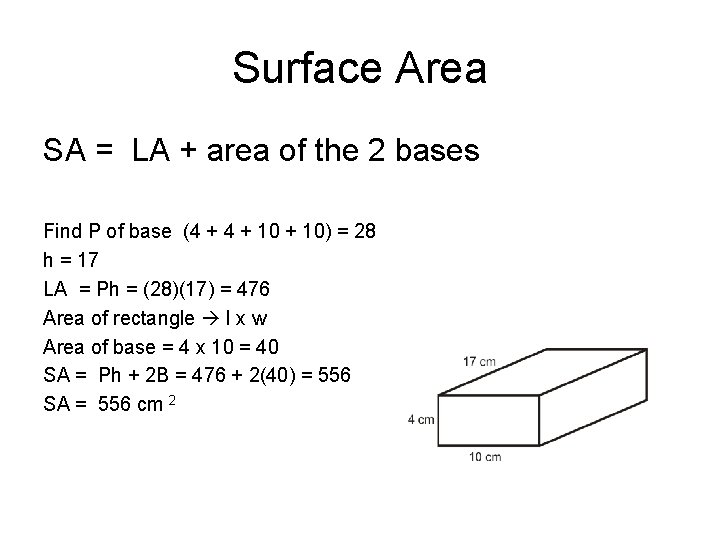Surface Area SA = LA + area of the 2 bases Find P of base (4 + 10) = 28 h = 17 LA = Ph = (28)(17) = 476 Area of rectangle l x w Area of base = 4 x 10 = 40 SA = Ph + 2 B = 476 + 2(40) = 556 SA = 556 cm 2Find the Area and Perimeter Side equals 7 in. Side measures 3. 5 cm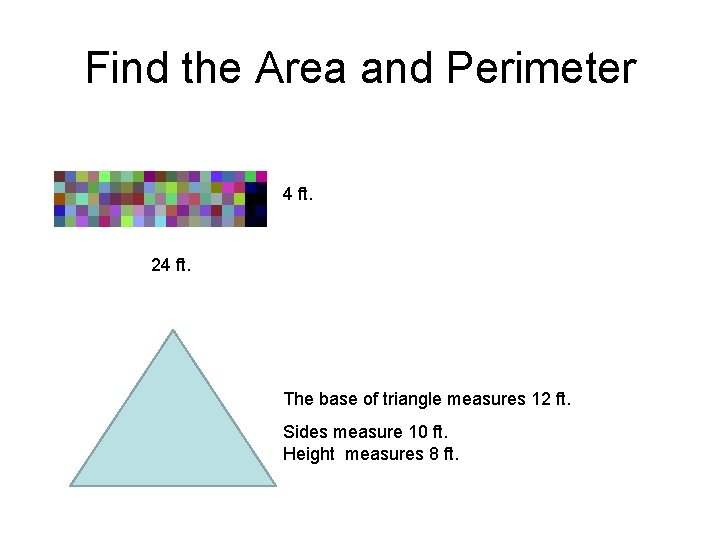Find the Area and Perimeter 4 ft. 24 ft. The base of triangle measures 12 ft. Sides measure 10 ft. Height measures 8 ft.